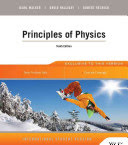### Physics

Data is displayed for academic year: 2023./2024.

#### Course Description

Through this calculus-based physics course students will be introduced to basics of mechanics, principles of Special theory of relativity, basics of electromagnetism, and wave optics. Topics in mechanics include particle kinematics and dynamics in three-dimensional space, principles of conservation of energy and momentum, oscillatory motion, and mechanical waves. Topics in electromagnetism cover Maxwell's equations and electromagnetic waves.

#### Study Programmes

[FER3-EN] Computing - study
(2. semester)
[FER3-EN] Electrical Engineering and Information Technology - study
(2. semester)

#### Learning Outcomes

1. Apply calculus techniques (derivative, integration) to analysis of physical problems.
2. Define kinematic variables (vectors of position, velocity, and acceleration) in three dimensional space and apply relationships between them.
3. Analyze simple mechanical systems and apply Newton's equations of motion.
4. Apply energy and momentum conservation principles to mechanical systems.
5. Apply basic principles (2. Newton's law) to complex phenomena in mechanics (harmonic oscillator, waves).
6. Explain principles of special theory of relativity.
7. Explain the laws of electromagnetism and apply them to simple physical situations.
8. Derive the wave equation for electromagnetic waves from Maxwell's equations.
9. Explain the phenomena of light interference and polarization.

#### Forms of Teaching

Lectures

Lectures with solved problems and experiments

Partial e-learning

Assignments through e-learning platform

Laboratory

Students perform laboratory experiments

Continuous Assessment Exam
Laboratory Exercises 5 % 10 % 5 % 10 %
Homeworks 0 % 10 % 0 % 10 %
Mid Term Exam: Written 0 % 28 % 0 %
Final Exam: Written 0 % 28 %
Final Exam: Oral 24 %
Exam: Written 0 % 56 %
Exam: Oral 24 %
##### Comment:

In the mid-term exam and in the final exam one (of four) problem must be correctly solved. In the written exam two (of six) problems must be correctly completed. In the oral part of the exam at least 8 (of 24) points.

#### Week by Week Schedule

1. Kinematics (reference frame, position, velocity and acceleration of the particle). Motion in the rectangular coordinate system. Galilean transformations of the position and velocity of a particle
2. First Newton's law. Inertial frame of reference. Particle momentum. 2nd Newton's law. Newton's equation of motion. Motion under constant force (free-fall, incline, pulleys)
3. Interaction of particles. 3rd Newton's law. Internal and external forces in a system of particles. Conservation of linear momentum in a system of particles
4. Definition of work and power. Kinetic energy. Conservative force. Stable and unstable equilibrium. Conservation of mechanical energy. Dissipative forces
5. Simple harmonic oscillator (mass on a spring). Equation of motion and the solution. Energy of oscillation. Damped oscillation: subcritical, critical and supercritical damping. Forced oscillations. Amplitude and phase. Resonance. Coupled oscillators. Normal modes of oscillation
6. Propagation of waves. Frequency and phase vector. Phase speed. Superposition. Wave equation and its solution. Wave packet. Transverse wave (equation of motion, speed of propagation, power, standing wave)
7. Transmission and reflexion of transversal waves. Coefficients of transmission and reflexion. Longitudinal wave (equation of motion, speed of propagation). Adiabatic sound (speed of propagation). Doppler effect
8. Midterm exam
9. Experimental background. Postulates of the Special Relativity. Relativistic kinematics. Lorentz transformations. Length contraction. Time dilation. Relativistic energy and momentum. Rest mass of a particle
10. Electrostatics; Coulomb force; Electric field and the potential, Magnetostatics; Magnetic field of a straight wirel Biot-Savart law
11. First Maxwell's equation. Gauss' law for the electric field. Second Maxwell's equation. Gauss' law for the magnetic field
12. Third Maxwell's equation. Faraday's law of induction. Fourth Maxwell's equation. Ampère-Maxwell law
13. Wave equation for the electromagnetic field. Plane wave solution to the wave equation. Properties of the plane waves. Linear and circular polarisation of electromagnetic radiation. Poynting's theorem and vector. Intensity of electromagnetic radiation. Energy density of electromagnetic field. Polarization of light. Malus law
14. Coherent sources. Constructive and destructive interference of two sources. Young's experiment. Phase shift due to reflection of radiation. Interference of light reflected on thin films, Interferometry. Detection and measurement of small displacements
15. Final exam

#### Literature

D. Horvat (2005.), Fizika 1: Mehanika i toplina, Hinus
D. Horvat (2011.), Fizika 2: titranje, valovi, elektromagnetizam, optika i uvod u modernu fiziku, Neodidakta
P. Kulišić (2005.), Mehanika i toplina, Školska knjiga
V. Henč-Bartolić, P. Kulišić (1991.), Valovi i optika, Školska knjiga
V. Henč-Bartolić, M. Baće, L. Bistričić, D. Horvat, P. Kulišić, Z. Narančić, T. Petković, D. Pevec (2002.), Riješeni zadaci iz valova i optike, Školska knjigaDavid Halliday, Robert Resnick, Jearl Walker (2014.), Principles of Physics, Wiley
V. Henč-Bartolić, M. Baće, L. Bistričić, D. Horvat, P. Kulišić, Z. Narančić, T. Petković, D. Pevec (1996.), Riješeni zadaci iz mehanike i topline, Školska knjiga
Saša Ilijić (2020.), Mehanika - pojmovi, načela i odabrani primjeri, http://sail.zpf.fer.hr/labs/mehanika2.pdf

#### General

ID 209627
Summer semester
6 ECTS
L1 English Level
L2 e-Learning
75 Lectures
0 Seminar
0 Exercises
15 Laboratory exercises
0 Project laboratory